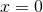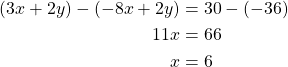# Review Systems of Equations

Where two numbers are unknown, we need two independent relations for those two numbers to calculate their respective values.

## Example:When graphed, both of these relations form straight lines, however the methods here are not restricted to linear equations.

Each equation gives new information about howandare related to each other.

Take one equation on its own:. There are infinitely many values ofandthat work in this equation – eg,and. There are infinitely many ordered pairsthat satisfy this equation.

However when graphed together, straight line graphs fall into one of three categories:

• parallel and never intersect – no common point;
• not parallel, intersect at one and only one point;
• parallel and always intersect – that is, the lines are coincident.

When graphed, it is obvious what category our set of equations fall into. However without the graph the algebra will let us know:

1. if they are parallel and don’t intersect, our algebra has a final line such aswhich makes no sense. This is a contradiction informing us that no values will work in both equations.
2. if they are not parallel and intersect at one and only one point, our algebra will result in the unique values ofandof this point.
3. if they are coincident, are algebra will end up with a linear relationship betweenand, such as– meaning that any point on this line is on both lines, all are solutions to the system. There are infinitely many solutions.

# Solve with SubstitutionFirst, let’s isolate eitherorin one or the other of the equations. In this case, it is easy to isolatein the second equation.Now we take the expression forin the second equation and substitute it to the first, as follows:Finally, substitute this value ofinto the equation(or the other equation) to find.Solution:,.

Check to see that this solution works in the both of our original equations.

# Solve with EliminationFirst, it is helpful to align the unknowns:Next, we examine to see if any unknown (eitheror) has the same coefficient in both equations. This is not true in our example.

We could:

• Multiply the first equation by 4 and the second equation by 3 to havein both equations, or
• Multiply the second equation by 2 to havein both equations.

The latter is more efficient. Multiplying the second equation by 2 gives:Subtract equation 2 from equation 1 to eliminate theterm:or, more simply:Now substitute this value ofinto the equation(or the other equation) to find.Solution:,.